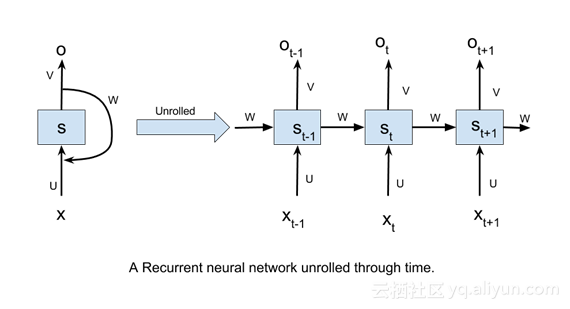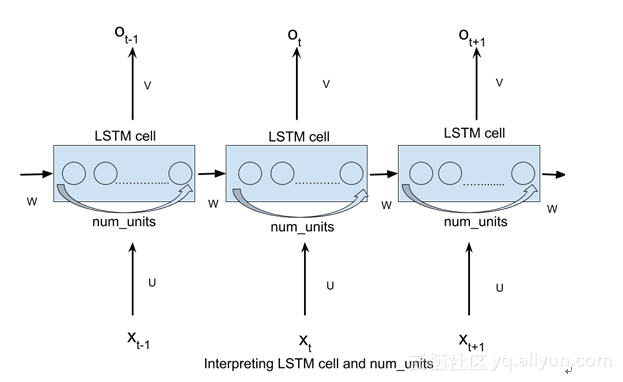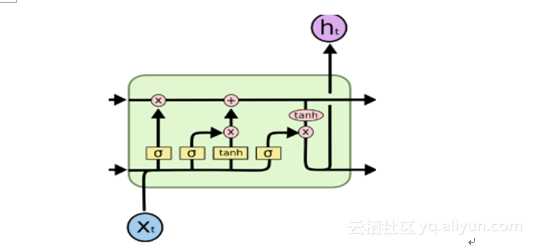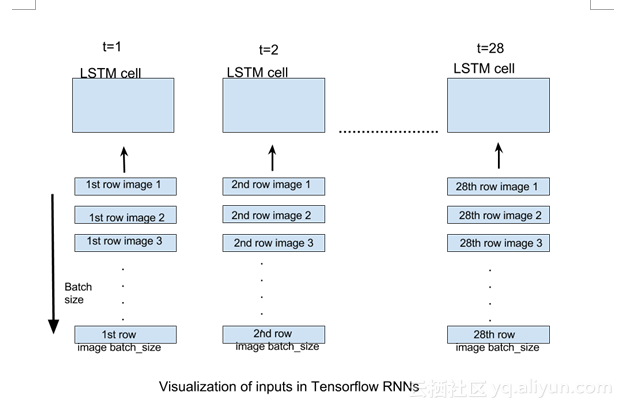# TensorFlow实现基本的LSTM网络

LSTM，全称为长短期记忆网络(Long Short Term Memory networks)，是一种特殊的RNN，能够学习到长期依赖关系。LSTM由Hochreiter & Schmidhuber (1997)提出，许多研究者进行了一系列的工作对其改进并使之发扬光大。

了解LSTM请前往——LSTM的“前生今世”

LSTM在解决许多问题上效果非常好，现在被广泛使用。它们主要用于处理序列数据。这个博客的主要目的是让读者了解在TensorFlow中，如何实现基本的LSTM网络并掌握实现的细节。为了实现这一目标，我们把MNIST作为我们的数据集。

MNIST数据集：

MNIST数据集由手写数字及其相应标签的图像组成。我们可以借助TensorFlow的内置功能下载和读取数据：

from tensorflow.examples.tutorials.mnist import input_data
mnist = input_data.read_data_sets("/tmp/data/", one_hot=True)

### 数据分为三部分：

LSTM通常用于解决复杂序列的相关问题，如NLP领域的实验：语言建模、字嵌入，编码器等。MNIST给了我们解决这类问题的机会。这里的输入数据只是一组像素值。我们可以轻松地格式化这些值，并集中应用到问题细节上。

A vanilla RNN1.Xt是指时间步长t的输入。

2.St是指时间步长t处的隐藏状态。它可以被可视化为网络的“内存”。

3.Ot指的是输出在时间步长t。

4.U，V和W是所有时间步长共享的参数。该参数共享的意义在于，我们的模型在不同输入的时间步长可以执行相同的任务。

1.TensorFlow中LSTM细胞的解释。

2.将输入格式化，然后将其输入到TensorFlow RNNs中。

TensorFlow中LSTM细胞的解释：

tf.contrib.rnn.BasicLSTMCell(num_units)

num_units也可以解释为前馈神经网络隐藏层的类比。前馈神经网络隐层中的节点num_units数目等于LSTM网络每个时间步长的LSTM单元的数量。以下图片应该可以帮助你理解：张量流（tensorflow）中最简单的RNN形式是在static_rnn中定义：

tf.static_rnn(cell,inputs)

当然还有其他形式的定义方法，这里我们只需要用到最简单的定义方法。

该inputs参数是为了接受形状张量列表[batch_size,input_size]。该列表的长度是网络展开的时间步长数，即该列表的每个元素对应于我们展开网络的相应时间步长的输入。

对于我们的MNIST图像的情况，我们有大小为28X28的图像。它们可以被推断为具有28行28像素的图像。我们将通过28个时间步骤展开我们的网络，使得在每个时间步长，我们可以输入一行28像素（input_size），从而通过28个时间步长输入完整的图像。如果我们提供batch_size图像的数量，每个时间步长将提供相应的batch_size图像行。下图应该可以解释上述描述：### Code

import tensorflow as tf
from tensorflow.contrib import rnn
#import mnist dataset
from tensorflow.examples.tutorials.mnist import input_data
mnist=input_data.read_data_sets("/tmp/data/",one_hot=True)
#define constants
#unrolled through 28 time steps
time_steps=28
#hidden LSTM units
num_units=128
#rows of 28 pixels
n_input=28
#learning rate for adam
learning_rate=0.001
#mnist is meant to be classified in 10 classes(0-9).
n_classes=10
#size of batch
batch_size=128

#weights and biases of appropriate shape to accomplish above task
out_weights=tf.Variable(tf.random_normal([num_units,n_classes]))
out_bias=tf.Variable(tf.random_normal([n_classes]))
#defining placeholders
#input image placeholder
x=tf.placeholder("float",[None,time_steps,n_input])
#input label placeholder
y=tf.placeholder("float",[None,n_classes])

#processing the input tensor from [batch_size,n_steps,n_input] to "time_steps" number of [batch_size,n_input] tensors
input=tf.unstack(x ,time_steps,1)

#defining the network
lstm_layer=rnn.BasicLSTMCell(n_hidden,forget_bias=1)
outputs,_=rnn.static_rnn(lstm_layer,input,dtype="float32")

#converting last output of dimension [batch_size,num_units] to [batch_size,n_classes] by out_weight multiplication
prediction=tf.matmul(outputs[-1],out_weights)+out_bias

#loss_function
loss=tf.reduce_mean(tf.nn.softmax_cross_entropy_with_logits(logits=prediction,labels=y))
#optimization
opt=tf.train.AdamOptimizer(learning_rate=learning_rate).minimize(loss)
#model evaluation
correct_prediction=tf.equal(tf.argmax(prediction,1),tf.argmax(y,1))
accuracy=tf.reduce_mean(tf.cast(correct_prediction,tf.float32))

#initialize variables
init=tf.global_variables_initializer()
with tf.Session() as sess:
sess.run(init)
iter=1
while iter<800:
batch_x,batch_y=mnist.train.next_batch(batch_size=batch_size)
batch_x=batch_x.reshape((batch_size,time_steps,n_input))
sess.run(opt, feed_dict={x: batch_x, y: batch_y})
if iter %10==0:
acc=sess.run(accuracy,feed_dict={x:batch_x,y:batch_y})
los=sess.run(loss,feed_dict={x:batch_x,y:batch_y})
print("For iter ",iter)
print("Accuracy ",acc)
print("Loss ",los)
print("__________________")
iter=iter+1

这里要注意的一个关键点，我们的图像基本上是被平坦化为一个单一的维度矢量784。函数next_batch(batch_size)必然返回batch_size784维度向量的批次，因此它们被重塑为[batch_size,time_steps,n_input]可以被占位符接受。

#calculating test accuracy
test_data = mnist.test.images[:128].reshape((-1, time_steps, n_input))
test_label = mnist.test.labels[:128]
print("Testing Accuracy:", sess.run(accuracy, feed_dict={x: test_data, y: test_label}))

这个博客目的是让读者对张量流中RNN的实现细节有所了解。以便我们建立了一些更复杂的模型，以有效地在张量流中使用RNN。

07-2310-177063
09-036万+
11-19
11-01
11-255万+
06-024444
12-222680
11-012万+
12-193万+
05-31699
09-11342
12-021万+
09-091万+
03-224405
©️2020 CSDN 皮肤主题: 大白 设计师:CSDN官方博客